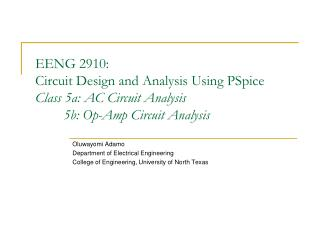DownloadDownload PresentationOluwayomi Adamo Department of Electrical Engineering

# Oluwayomi Adamo Department of Electrical Engineering

Download Presentation## Oluwayomi Adamo Department of Electrical Engineering

- - - - - - - - - - - - - - - - - - - - - - - - - - - E N D - - - - - - - - - - - - - - - - - - - - - - - - - - -
##### Presentation Transcript

1. EENG 2910: Circuit Design and Analysis Using PSpiceClass 5a: AC Circuit Analysis 5b: Op-Amp Circuit Analysis Oluwayomi Adamo Department of Electrical Engineering College of Engineering, University of North Texas

2. 5a. New Parts Needed for AC Analysis • AC Sources • VAC and IAC • Both are form “source.olb” • The ACMAG and ACPHASE properties can be configured in the Property Editor. • For AC sources, you can also set DC value. But for AC analysis, you can set DC value to zero. • The frequency f of the AC source is configured in Simulation Profile Editor. The frequency needs to be in the Hz unit. • The simulation type for AC analysis is AC Sweep/Noise. • Printer Parts • IPRINT is used to print current into the output file. • VPRINT1 (with one lead) and VPRINT2 (with two lead) are used to print voltage into the output file. • Both IPRINT and VPRINT are from “special.olb” • In Property Editor you can configure to select which property to print. For example, REAL, IMAG, MAG, PHASE. Put “y” for a property, if you want to print that property. • If one of the fields does not appear in the property editor for some of the printers, add it by pressing the “New Column …” button in the property editor. EENG 2910, Class 5

3. New Command Needed in PSpice AD • To plot magnitude • V( ) or VM( ) and I() or IM( ) for peak value in linear scale • VDB( ) and IDB in dB scale • To plot phase • VP( ) and IP( ) for phase • To plot real part and imaginary part • VR( ), VI( ), IR( ), II( ) EENG 2910, Class 5

4. Coupled Inductors • The symbol for mutual coupling is K. • The mutual coupling part is the “K-Linear” from “analog.olb”. • K-Linear can couple up to eight inductors. • The mutual coupling K couples two or more inductors with a coupling coefficient k. • The coupling coefficient k: • PSpice assumes a dot on the first node of each inductor. • Read the book, page 145, for more details. M is mutual inductance EENG 2910, Class 5

5. Example 5.2 Figure 5.2.1 Figure 5.2.2 EENG 2910, Class 5

6. Example 5.4 The AC source is VSIN Figure 5.4.1 Both of these vertical lines are dashed lines, not wires! Figure 5.4.2 EENG 2910, Class 5

7. 5b. Op Amps Circuit Example 10.2 (Your final drawing of circuit schematics) Figure 10.2.1 Figure 10.2.2 Question: Please explain the relation between V(V1:+) and V(out). EENG 2910, Class 5

8. Example 10.4 (Your final drawing of circuit schematics) Figure 10.4.1 Figure 10.4.2 EENG 2910, Class 5

9. Assignment 5 • Repeat and reproduce the results for Examples 5.2, and 5.4. • Repeat and reproduce the results for Examples 10.2, and 10.4, EENG 2910, Class 5